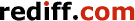Print this article

# The number's up for 'Pi', say mathematicians

June 28, 2011 15:47 IST

The humble "pi" may have had its day. Mathematicians are campaigning for the most important number in the world to be replaced with alternate value "tau".

They are claiming that the number -- the constant which references the circumference of a circle to its diameter -- is wrong and it should be replaced with tau, the Daily Mail reported.

The mathematicians have said that while 3.14159265, the number's value, is not incorrect, it is the wrong figure to be associated with the properties of a circle as a matter of course. Tau has a value twice that of pi of 6.28.

"For all these years, we have been looking at the wrong number when we have been looking at pi. Pi simply isn't the most natural number that we should associate with a circle. The proper number is 2pi, or tau," Kevin Houston at the University of Leeds told The Times newspaper.

The number has long been seen as being essential to many mathematical formulae as well as being vital to equations in science and engineering. It is used when calculating the circumference of a circle by multiplying the diameter by the value of pi, while its area can be deduced by multiplying pi by the square of its radius.

However, mathematicians campaigning for its replacement argue that since so many formulae require the use of tau that may be used as the main circle constant instead.

"Mathematicians don't measure angles in degrees, we measure them in radians, and there are 2pi radians in a circle. That leads to all sorts of unnecessary confusion. If you take a quarter of a circle, it has a quarter of 2pi radians, or half pi.

"For the number of radians in three quarters of a circle, you have to think about it. It doesn't come naturally. How much simpler it'd be if we just used tau instead of pi. The circle would have tau radians, a semicircle would have half tau, a quarter of a circle a quarter tau, and so on. You don't have to think," Dr Houston said.

Pi -- taken from the first letter of the Greek word for "perimeter" -- was first given its name in 1706 by mathematician William Jones. Both the Ancient Egyptians and the Babylonians were believed to have known an approximate value of pi, while a version also appears in Bible.

© Copyright 2019 PTI. All rights reserved. Republication or redistribution of PTI content, including by framing or similar means, is expressly prohibited without the prior written consent.